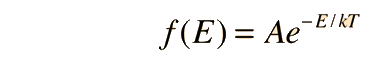# Fractional Population of Excited States

Fundamental to our understanding of classical molecular phenomena is the Boltzmann distribution, which tells us that the probability that any one molecule will be found with energy E decreases exponentially with energy; i.e., any one molecule is highly unlikely to grab much more than its average share of the total energy available to all the molecules. Mathematically, the Boltzmann distribution can be written in the formApplications

The fractional population of an excited state can be calculated from the Boltzmann distribution. The distribution of energy depends upon the distribution function and the density of available states for a given energy level. In many cases, there will be more ways to achieve an upper energy level, so that statistical weight must be taken into account in calculating populations.

The example here will not take into account the density of states, but will consider the case of a collection of point particles at thermal equilibrium at temperature T. This can give some insight into the probability of reaching some threshold for a physical phenomenon, or the probability of overcoming some barrier. Note that this is a classical calculation and does not take into account the possibility of quantum mechanical tunneling.

For temperature
T = C = F = K = x10^ K,

the fraction of them which will have energy above the threshold
E = eV = MeV = GeV
E = x 10^ joules = x10^ eV.

will be

e-E/kT = = x10^ .

Calculation notes: Default values will be entered for unspecified parameters, but all values can be changed. Default values will be substituted if you put in a fraction greater than one. Otherwise, entering a fraction will cause the temperature to be calculated for the given energy.

 Average thermal energy
Index

Kinetic theory concepts

 HyperPhysics***** Thermodynamics R Nave
Go Back